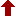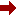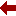#### SLIM21

 Sort Order Chronological Author Title ItemType,Author ItemType,Title Display Format Tabular Publication Bibliography Items / Page 5 10 25

Click the serial number on the left to view the details of the item.
#  AuthorTitleAccn#YearItem Type Claims
1 Kantorovich, Lev Mathematics for Natural Scientists I12528 2022 Book
2 Barber, J. R Elasticity I12472 2022 Book
3 Steinhauser, Martin Oliver Computational Multiscale Modeling of Fluids and Solids I12437 2022 Book
4 Cacuci, Dan Gabriel The nth-Order Comprehensive Adjoint Sensitivity Analysis Methodology, Volume I I12435 2022 Book
5 Bonamente, Massimiliano Statistics and Analysis of Scientific Data I12430 2022 Book
6 Huerta Cu??llar, Guillermo Complex Systems and Their Applications I12413 2022 Book
7 Volchenkov, Dimitri Mathematical Methods in Modern Complexity Science I12384 2022 Book
8 Tang, Kwong-Tin Mathematical Methods for Engineers and Scientists 1 I12284 2022 Book
9 Milstein, Grigori N Stochastic Numerics for Mathematical Physics I11924 2021 eBook
10 Derbel, Nabil Advanced Methods for Human Biometrics I11791 2021 eBook
 (page:1 / 2) [#14] Next Page Last Page

1.TitleMathematics for Natural Scientists : Fundamentals and Basics
Author(s)Kantorovich, Lev
PublicationCham, 1. Imprint: Springer 2. Springer International Publishing, 2022.
DescriptionXXIII, 768 p. 189 illus., 163 illus. in color : online resource
Abstract NoteThis book, now in a second revised and enlarged edition, covers a course of mathematics designed primarily for physics and engineering students. It includes all the essential material on mathematical methods, presented in a form accessible to physics students and avoiding unnecessary mathematical jargon and proofs that are comprehensible only to mathematicians. Instead, all proofs are given in a form that is clear and sufficiently convincing for a physicist. Examples, where appropriate, are given from physics contexts. Both solved and unsolved problems are provided in each section of the book. The second edition includes more on advanced algebra, polynomials and algebraic equations in significantly extended first two chapters on elementary mathematics, numerical and functional series and ordinary differential equations. Improvements have been made in all other chapters, with inclusion of additional material, to make the presentation clearer, more rigorous and coherent, and the number of problems has been increased at least twofold. Mathematics for Natural Scientists: Fundamentals and Basics is the first of two volumes. Advanced topics and their applications in physics are covered in the second volume the second edition of which the author is currently being working on
ISBN,Price9783030912222
Keyword(s)1. Applications of Mathematics 2. Chemometrics 3. Diseases 4. EBOOK 5. EBOOK - SPRINGER 6. ENGINEERING MATHEMATICS 7. Engineering???Data processing 8. Mathematical and Computational Engineering Applications 9. Mathematical Applications in Chemistry 10. Mathematical Methods in Physics 11. MATHEMATICAL PHYSICS 12. MATHEMATICS 13. Theoretical, Mathematical and Computational Physics
Item TypeBook
Circulation Data
 Accession# Call# Status Issued To Return Due On Physical Location I12528 On Shelf

2.TitleElasticity
Author(s)Barber, J. R
PublicationCham, 1. Imprint: Springer 2. Springer International Publishing, 2022.
DescriptionXX, 637 p. 131 illus., 2 illus. in color : online resource
Abstract NoteThis book emphasizes engineering applications of elasticity. This is a first-year graduate textbook in linear elasticity. It is written with the practical engineering reader in mind, dependence on previous knowledge of solid mechanics, continuum mechanics or mathematics being minimized. Examples are generally worked through to final expressions for the stress and displacement fields in order to explore the engineering consequences of the results. This 4th edition presents new and revised material, notably on the Eshelby inclusion problem and anisotropic elasticity. The topics covered are chosen with a view to modern research applications in fracture mechanics, composite materials, tribology and numerical methods. Thus, significant attention is given to crack and contact problems, problems involving interfaces between dissimilar media, thermoelasticity, singular asymptotic stress fields and three-dimensional problems
ISBN,Price9783031152146
Keyword(s)1. Applications of Mathematics 2. Classical and Continuum Physics 3. EBOOK 4. EBOOK - SPRINGER 5. Engineering Fluid Dynamics 6. ENGINEERING MATHEMATICS 7. Engineering???Data processing 8. FLUID MECHANICS 9. Mathematical and Computational Engineering Applications 10. MATHEMATICS 11. MECHANICAL ENGINEERING 12. Mechanics, Applied 13. PHYSICS 14. Solid Mechanics 15. SOLIDS
Item TypeBook
Circulation Data
 Accession# Call# Status Issued To Return Due On Physical Location I12472 On Shelf

3.TitleComputational Multiscale Modeling of Fluids and Solids : Theory and Applications
Author(s)Steinhauser, Martin Oliver
PublicationCham, 1. Imprint: Springer 2. Springer International Publishing, 2022.
DescriptionXXVII, 432 p. 145 illus., 55 illus. in color : online resource
Abstract NoteThe expanded 3rd edition of this established textbook offers an updated overview and review of the computational physics techniques used in materials modelling over different length and time scales. It describes in detail the theory and application of some of the most important methods used to simulate materials across the various levels of spatial and temporal resolution. Quantum mechanical methods such as the Hartree-Fock approximation for solving the Schr??dinger equation at the smallest spatial resolution are discussed as well as the Molecular Dynamics and Monte-Carlo methods on the micro- and meso-scale up to macroscopic methods used predominantly in the Engineering world such as Finite Elements (FE) or Smoothed Particle Hydrodynamics (SPH). Extensively updated throughout, this new edition includes additional sections on polymer theory, statistical physics and continuum theory, the latter being the basis of FE methods and SPH. Each chapter now first provides an overview of the key topics covered, with a new ???key points??? section at the end. The book is aimed at beginning or advanced graduate students who want to enter the field of computational science on multi-scales. It provides an in-depth overview of the basic physical, mathematical and numerical principles for modelling solids and fluids on the micro-, meso-, and macro-scale. With a set of exercises, selected solutions and several case studies, it is a suitable book for students in physics, engineering, and materials science, and a practical reference resource for those already using materials modelling and computational methods in their research
ISBN,Price9783030989545
Keyword(s)1. Characterization and Analytical Technique 2. Computational Mathematics and Numerical Analysis 3. EBOOK 4. EBOOK - SPRINGER 5. ENGINEERING MATHEMATICS 6. Engineering???Data processing 7. GEOLOGY 8. Materials???Analysis 9. Mathematical and Computational Engineering Applications 10. MATHEMATICAL PHYSICS 11. Mathematics???Data processing 12. Theoretical, Mathematical and Computational Physics
Item TypeBook
Circulation Data
 Accession# Call# Status Issued To Return Due On Physical Location I12437 On Shelf

4.TitleThe nth-Order Comprehensive Adjoint Sensitivity Analysis Methodology, Volume I : Overcoming the Curse of Dimensionality: Linear Systems
Author(s)Cacuci, Dan Gabriel
PublicationCham, 1. Imprint: Springer 2. Springer International Publishing, 2022.
DescriptionXII, 362 p : online resource
ISBN,Price9783030963644
Keyword(s)1. Computational Physics and Simulations 2. COMPUTER SIMULATION 3. EBOOK 4. EBOOK - SPRINGER 5. Energy and state 6. Energy policy 7. Energy Policy, Economics and Management 8. ENGINEERING MATHEMATICS 9. Engineering???Data processing 10. Mathematical and Computational Engineering Applications 11. Mathematical Modeling and Industrial Mathematics 12. MATHEMATICAL MODELS 13. MATHEMATICAL PHYSICS 14. NUCLEAR PHYSICS 15. Statistical Theory and Methods 16. Statistics??
Item TypeBook
Circulation Data
 Accession# Call# Status Issued To Return Due On Physical Location I12435 On Shelf

5.TitleStatistics and Analysis of Scientific Data
Author(s)Bonamente, Massimiliano
PublicationSingapore, 1. Imprint: Springer 2. Springer Nature Singapore, 2022.
DescriptionXXIII, 488 p. 58 illus., 48 illus. in color : online resource
Abstract NoteThis book is the third edition of a successful textbook for upper-undergraduate and early graduate students, which offers a solid foundation in probability theory and statistics and their application to physical sciences, engineering, biomedical sciences and related disciplines. It provides broad coverage ranging from conventional textbook content of probability theory, random variables, and their statistics, regression, and parameter estimation, to modern methods including Monte-Carlo Markov chains, resampling methods and low-count statistics. In addition to minor corrections and adjusting structure of the content, particular features in this new edition include: Python codes and machine-readable data for all examples, classic experiments, and exercises, which are now more accessible to students and instructors New chapters on low-count statistics including the Poisson-based Cash statistic for regression in the low-count regime, and on contingency tables and diagnostic testing. An additional example of classic experiments based on testing data for SARS-COV-2 to demonstrate practical applications of the described statistical methods. This edition inherits the main pedagogical method of earlier versions???a theory-then-application approach???where emphasis is placed first on a sound understanding of the underlying theory of a topic, which becomes the basis for an efficient and practical application of the materials. Basic calculus is used in some of the derivations, and no previous background in probability and statistics is required. The book includes many numerical tables of data as well as exercises and examples to aid the readers' understanding of the topic
ISBN,Price9789811903656
Keyword(s)1. Applied Statistics 2. Data Analysis and Big Data 3. EBOOK 4. EBOOK - SPRINGER 5. ENGINEERING MATHEMATICS 6. Engineering???Data processing 7. Mathematical and Computational Engineering Applications 8. Mathematical Methods in Physics 9. MATHEMATICAL PHYSICS 10. Mathematical statistics???Data processing 11. Quantitative research 12. Statistical Theory and Methods 13. Statistics and Computing 14. Statistics??
Item TypeBook
Circulation Data
 Accession# Call# Status Issued To Return Due On Physical Location I12430 On Shelf

6.TitleComplex Systems and Their Applications : Second International Conference (EDIESCA 2021)
Author(s)Huerta Cu??llar, Guillermo;Campos Cant??n, Eric;Tlelo-Cuautle, Esteban
PublicationCham, 1. Imprint: Springer 2. Springer International Publishing, 2022.
DescriptionX, 269 p. 149 illus., 129 illus. in color : online resource
Abstract NoteThis book is a compilation of scientific articles written by recognized researchers, and select students, participating in the Second Conference on the Study of Complex Systems and their Applications (EDIESCA 2021). EDIESCA 2021 arose from the need for academic and research groups that carry out this scientific research to disseminate their results internationally. The study and characterization of systems with non-linear and/or chaotic behavior has been of great interest to researchers around the world, for which many important results have been obtained with various applications. The dynamic study of chaotic oscillators of different models, such as R??ssler, Lorenz, and Chua, has generated important advances in understanding of chemical reactions, meteorological behavior, design of electronic devices, and other applications. Topics at the event included applications for communications systems by masking techniques, financial behavior, networks analysis, nonlinear lasers, numerical modeling, electronic design, and other interesting topics in the area of complex systems. Additionally, there are results on numerical simulation and electronic designs to generate complex dynamic behaviors
ISBN,Price9783031024726
Keyword(s)1. Applied Dynamical Systems 2. COMPLEX SYSTEMS 3. DYNAMICS 4. EBOOK 5. EBOOK - SPRINGER 6. ELECTRONIC CIRCUIT DESIGN 7. Electronics Design and Verification 8. ENGINEERING DESIGN 9. ENGINEERING MATHEMATICS 10. Engineering???Data processing 11. Mathematical and Computational Engineering Applications 12. Mechanics, Applied 13. Multibody systems 14. Multibody Systems and Mechanical Vibrations 15. NONLINEAR THEORIES 16. SYSTEM THEORY 17. VIBRATION
Item TypeBook
Circulation Data
 Accession# Call# Status Issued To Return Due On Physical Location I12413 On Shelf

7.TitleMathematical Methods in Modern Complexity Science
PublicationCham, 1. Imprint: Springer 2. Springer International Publishing, 2022.
DescriptionX, 197 p. 94 illus., 69 illus. in color : online resource
Abstract NoteThis book presents recent developments in nonlinear and complex systems. It provides recent theoretic developments and new techniques based on a nonlinear dynamical systems approach that can be used to model and understand complex behavior in nonlinear dynamical systems. It covers information theory, relativistic chaotic dynamics, data analysis, relativistic chaotic dynamics, solvability issues in integro-differential equations, and inverse problems for parabolic differential equations, synchronization and chaotic transient. Presents new concepts for understanding and modeling complex systems
ISBN,Price9783030794125
Keyword(s)1. Applied Dynamical Systems 2. Computational Intelligence 3. DYNAMICS 4. EBOOK 5. EBOOK - SPRINGER 6. ENGINEERING MATHEMATICS 7. Engineering???Data processing 8. Mathematical and Computational Engineering Applications 9. MATHEMATICAL PHYSICS 10. NONLINEAR THEORIES
Item TypeBook
Circulation Data
 Accession# Call# Status Issued To Return Due On Physical Location I12384 On Shelf

8.TitleMathematical Methods for Engineers and Scientists 1 : Complex Analysis and Linear Algebra
Author(s)Tang, Kwong-Tin
PublicationCham, 1. Imprint: Springer 2. Springer International Publishing, 2022.
DescriptionXV, 492 p. 129 illus : online resource
Abstract NotePart 1 of this popular graduate-level textbook focuses on mathematical methods involving complex analysis, determinants, and matrices, including updated and additional material covering conformal mapping. The second edition comes with extensive updates and additions, making them a more complete reference for graduate science and engineering students while imparting comfort and confidence in using advanced mathematical tools in both upper-level undergraduate and beginning graduate courses. This set of student-centered textbooks presents topics such as complex analysis, matrix theory, vector and tensor analysis, Fourier analysis, integral transformations, and ordinary and partial differential equations in a discursive style that is clear, engaging, and easy to follow. Replete with pedagogical insights from an author with more than 30 years of experience in teaching applied mathematics, this indispensable set of books features numerous clearly stated and completely worked out examples together with carefully selected problems and answers that enhance students' understanding and analytical skills
ISBN,Price9783031056789
Keyword(s)1. EBOOK 2. EBOOK - SPRINGER 3. ENGINEERING MATHEMATICS 4. Engineering???Data processing 5. Mathematical and Computational Engineering Applications 6. Mathematical Methods in Physics 7. MATHEMATICAL PHYSICS 8. PROJECTIVE GEOMETRY 9. Theoretical, Mathematical and Computational Physics
Item TypeBook
Circulation Data
 Accession# Call# Status Issued To Return Due On Physical Location I12284 On Shelf

9.TitleStochastic Numerics for Mathematical Physics
Author(s)Milstein, Grigori N;Tretyakov, Michael V
PublicationCham, Springer International Publishing, 2021.
DescriptionXXV, 736 p. 33 illus : online resource
Abstract NoteThis book is a substantially revised and expanded edition reflecting major developments in stochastic numerics since the first edition was published in 2004. The new topics, in particular, include mean-square and weak approximations in the case of nonglobally Lipschitz coefficients of Stochastic Differential Equations (SDEs) including the concept of rejecting trajectories; conditional probabilistic representations and their application to practical variance reduction using regression methods; multi-level Monte Carlo method; computing ergodic limits and additional classes of geometric integrators used in molecular dynamics; numerical methods for FBSDEs; approximation of parabolic SPDEs and nonlinear filtering problem based on the method of characteristics. SDEs have many applications in the natural sciences and in finance. Besides, the employment of probabilistic representations together with the Monte Carlo technique allows us to reduce the solution of multi-dimensional problems for partial differential equations to the integration of stochastic equations. This approach leads to powerful computational mathematics that is presented in the treatise. Many special schemes for SDEs are presented. In the second part of the book numerical methods for solving complicated problems for partial differential equations occurring in practical applications, both linear and nonlinear, are constructed. All the methods are presented with proofs and hence founded on rigorous reasoning, thus giving the book textbook potential. An overwhelming majority of the methods are accompanied by the corresponding numerical algorithms which are ready for implementation in practice. The book addresses researchers and graduate students in numerical analysis, applied probability, physics, chemistry, and engineering as well as mathematical biology and financial mathematics
ISBN,Price9783030820404
Keyword(s)1. BIOMATHEMATICS 2. Chemometrics 3. Computational Science and Engineering 4. EBOOK 5. EBOOK - SPRINGER 6. ENGINEERING MATHEMATICS 7. Engineering???Data processing 8. Mathematical and Computational Biology 9. Mathematical and Computational Engineering Applications 10. Mathematical Applications in Chemistry 11. MATHEMATICAL PHYSICS 12. Mathematics in Business, Economics and Finance 13. Mathematics???Data processing 14. Social sciences???Mathematics 15. Theoretical, Mathematical and Computational Physics
Item TypeeBook
Circulation Data
 Accession# Call# Status Issued To Return Due On Physical Location I11924 On Shelf

10.